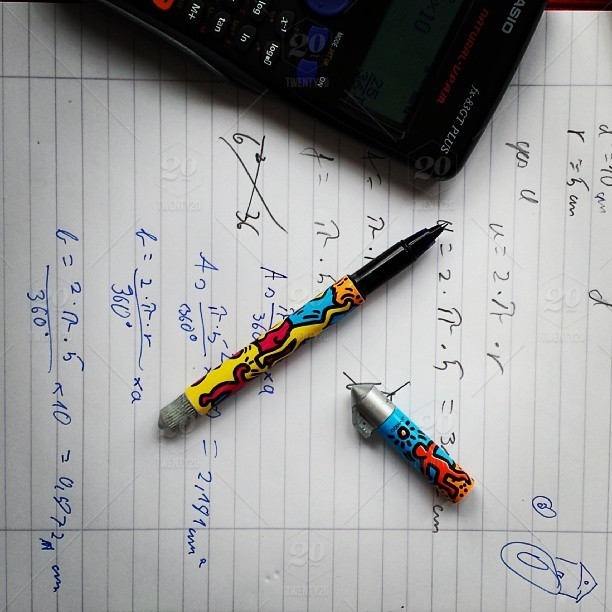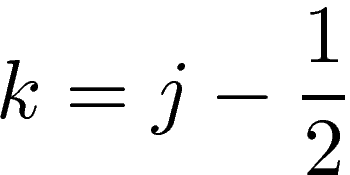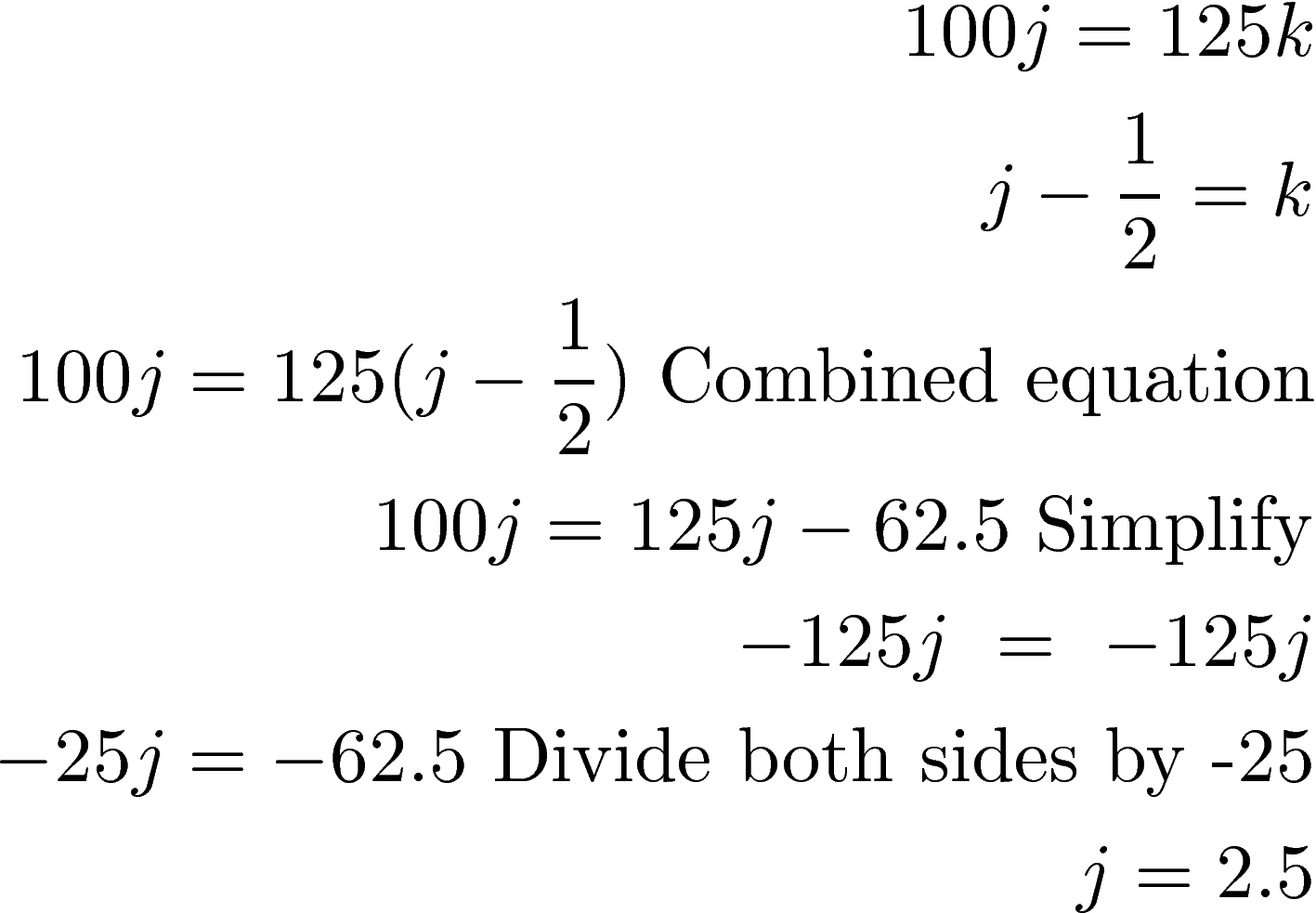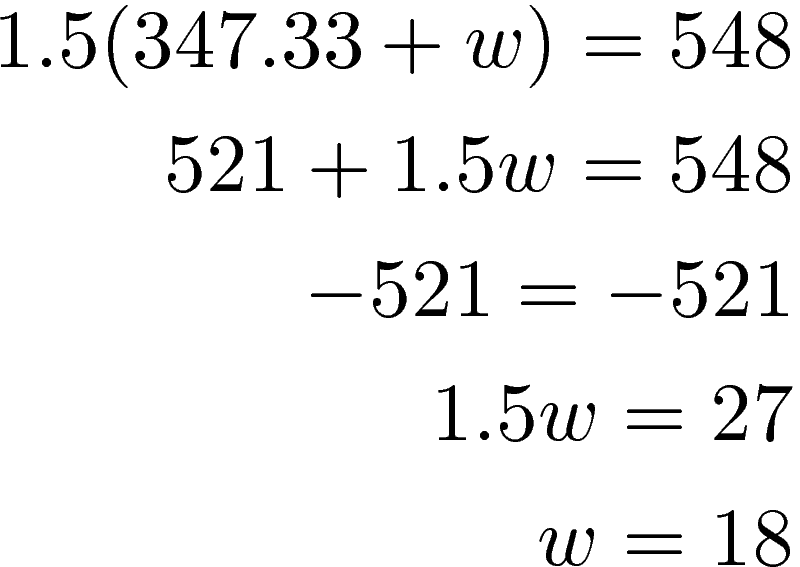# System of Equations and Uniform MotionThis post will provide examples of the use of a system of equations to solve uniform motion applications. A system of equations is used to solve for more than one variable. In the context of uniform motion, the basic equation is as follows

distance  = rate * time

We will look at the following examples

• Two objectives moving in the same direction

Objects Moving in the Same Directions

Below is the problem followed by the solution.

Dan leaves home and travels to Springfield at 100 kph. About 30 minutes later Sue leaves the house and also travels the same way to Springfield driving 125 kph. How long will it take Sue to catch Dan?

The easiest way to solve this is to create a table with all of the information we have. The table is below.

Names Rate * Time = Distance
Dan 100 j 100j
Sue 125 k 125k

We first need to recognize that they will drive the same distance this leads to one of our equationsHowever, we are not done. We also need to realize that Sue leaves half an hour later, which leads to the second equationWe can now solve our system of equationsWe know Dan travels for 2.5 hours before Sue catches him but we need to determine how long Sue drives before she catches Dan. We will take our answer J and plug it into the original equation for k.It will take Sue 2 hours to catch up with Dan.

In transportation, it is common for a plan or ship to be able to travel faster with a tailwind or downstream than with a headwind or upstream The example below shows you how to determine the speed needed to travel a certain distance in the same amount of time as well as the speed of the wind/current.

A plane can travel 548 miles in 1.5 hours with a tailwind but only 494 hours when flying into a headwind. Find the speed of the plane and the wind.

We will have two variables because there are two things we want to know

• p = the speed of the plane
• w = the speed of the wind

The tailwind makes the plane go faster, therefore, the speed of the plane will be the plane speed + the wind speed

The tailwind slows the plane down. Therefore, the tailwind will be the speed of the plane minus the windspeed.

Below is a table with all of the available information

Rate * Time = Distance
Tailwind p + w 1.5 548
Headwind p – w 1.5 494

The initial system of equations is as followsTo solve this system of equations we will use the elimination method as shown below.The plane travels 347.33 mph. We now take the value of p plug it into one of our equations to find the speed of the wind.The speed of the wind is 18 mph. We know the plane travels 347 + 18 = 365 mph with a tailwind and 347-18 = 329mph with a headwind.

Conclusion

A system of equations is proven to have a practical application. The assumption of a uniform speed is somewhat unrealistic in most instances. However, this assumption simplifies the calculation and prepares us for more complex models in the future.